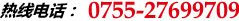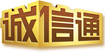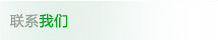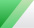## News CentersPhone：0755-27699709
Fax：0755-27448637
Service Hotline：18922801145
Contact Person：Mr. Li 13590397452
E-mail :szcjr@126.com
Website：www.szcjr.com
Address：Wai Park Building B, solid Xu Huafeng Industrial Zone, Bao'an District, Shenzhen
Company NewsHome > News Centers > Company News

## The working principle of the triode is how?

2013-12-20 20:46:08

The following analysis only for NPN type silicon transistor. As shown above, we put the current from the base B flow to the emitter of E called the base current Ib; the current collector to emitter flow from C E called the collector current Ic. The two current directions are out of the emitter, the emitter E with an arrow to indicate the direction of the current. Triode amplification is: control the collector current by the base current (assuming power can be supplied to the collector current large enough words), and small changes in base current, can cause large changes in collector current, and the change in a certain proportion: changes in the amount of collector current is beta times base current changes the amount of current change, which is amplified beta times, so we put the beta is called the transistor amplification (β is far greater than the general 1, for example, dozens, hundreds of). If we will be a change of small signal is added to the base to emitter, this will cause the change of the base current of Ib, the changes of Ib were amplified, resulted in changes in the Ic big. If the collector current Ic is through a resistor R, then according to the voltage calculation formula of U=R*I can calculate, change voltage will this resistor. We will be the voltage across this resistor is taken out, get the amplified voltage signal

Triode amplifying circuit used in practice, still need to add suitable bias circuit. There are several reasons for this. The first is due to the nonlinear transistor BE node (the equivalent of a diode), base current must be produced only on the input voltage to a certain degree (for the silicon tube, usually take 0.7V). When the voltage between the base and emitter base current is less than 0.7V, you can think of is 0. But the actual signal to amplified tend to be much smaller than the 0.7V, if not offset signal words, so small was not enough to cause the change of base current (because of less than 0.7V, the base current is 0). If we had with the last suitable current in the base electrode of the triode (called on the bias current, above the resistance Rb is used to provide the current, so it is called the base bias resistor), then when a small signal with the bias current superimposed together, small signal will lead to changes in the base current, and the change of the base current, will be amplified and output at the collector. Another reason is that the output signal range requirements, if not biased, so only to those who increase signal amplification, but not in reduced signal (because there is no bias when the collector current is 0, no longer reduces). But with bias, prior to a current collector, when the base current input variable hours, collector current can be reduced; when the base current input increases, the collector current is increased. This reduces the signal and increase the signal can be amplified.

Here to talk about saturated triode. Like the above graph, because of resistance limit of Rc (Rc is a fixed value, then the maximum current is U/Rc, where U is the power supply voltage), the collector current is not infinitely increase. With the increase of the base current, cannot make the collector current continues to increase, the transistor will enter saturated state. The general judgment triode saturation criterion is: Ib* β 'Ic. After entering the saturated state, the voltage between the triode collector to emitter will be very small, can be understood as a switch is closed. So we can take the transistor as switch: when the base current is 0, the transistor collector current is 0 (this is called the transistor cut-off), equivalent to switch off; when the base current is large, so that the transistor saturation, the equivalent of the switch. If the triode mainly work in the saturated state, then such a triode we usually call it switches

If we in the above diagram, the resistance of Rc to a light bulb, so when the base current is 0, the collector current is 0, the bulb out. If the base current is large (greater than through the lamp current divided by the transistor amplification beta), a triode is saturated, the equivalent of the switch is closed, the light bulb. Because the current control only need than lighting current beta of a point on the line, so we can control a large current through a small current passing. If the base current from 0 increase slowly, the brightness will increase as the (before the tube not saturated in triode).

<< Home